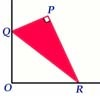#### You may also like### Baby Circle

A small circle fits between two touching circles so that all three circles touch each other and have a common tangent? What is the exact radius of the smallest circle?### Kissing

Two perpendicular lines are tangential to two identical circles that touch. What is the largest circle that can be placed in between the two lines and the two circles and how would you construct it?### Set Square

A triangle PQR, right angled at P, slides on a horizontal floor with Q and R in contact with perpendicular walls. What is the locus of P?

# Fixing It

##### Age 16 to 18Challenge Level

$A$ and $B$ are two fixed points on a circle and $RS$ is a variable diamater. What is the locus of the intersection $P$ of $AR$ and $BS$?